Tamilnadu State Board New Syllabus Samacheer Kalvi 12th Maths Guide Pdf Chapter 11 Probability Distributions Ex 11.2 Textbook Questions and Answers, Notes.

Tamilnadu Samacheer Kalvi 12th Maths Solutions Chapter 11 Probability Distributions Ex 11.2

Question 1.
Three fair coins are tossed simultaneously. Find the probability mass function for a number of heads that occurred.
Solution:
When three coins are tossed, the sample space is
S = {HHH, HHT, HTH, HTT, THH, THT, TTH, TTT}
‘X’ is the random variable that denotes the number of heads.
∴ ‘X’ can take the values of 0, 1, 2 and 3Sample space S = {HHH, HHT, HTH, THH, HTT, THT, TTH, TTT}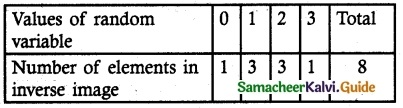Probability mass function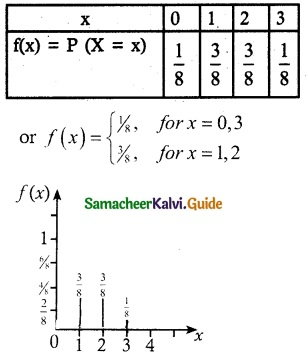Question 2.
A six sided die is marked ‘1’ on one face, ‘3’ on two of its faces and ‘5’ on remaining three faces. The die is thrown twice. If X denotes the total score in two throws, find
(i) the probability mass function
(ii) the cumulative distribution function
(iii) P(4 ≤ X < 10)
(iv) P(X ≥ 6)
Solution:
Let X be the random variable denotes the total score in two thrown of a die.
Sample space S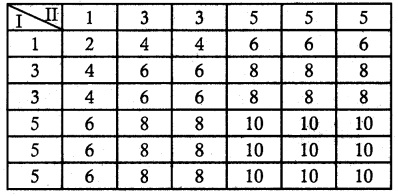n (S) = 36
X = {2, 4, 6, 8, 10}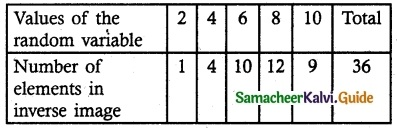(i) Probability mass function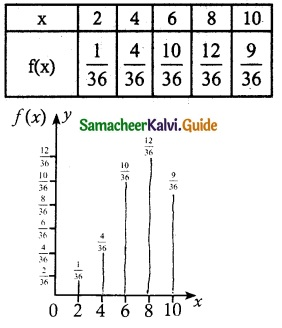(ii) Cumulative distribution function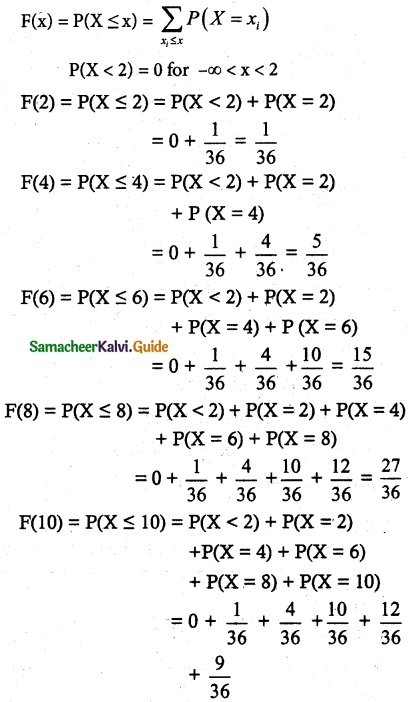= $$\frac { 36 }{ 36 }$$
= 1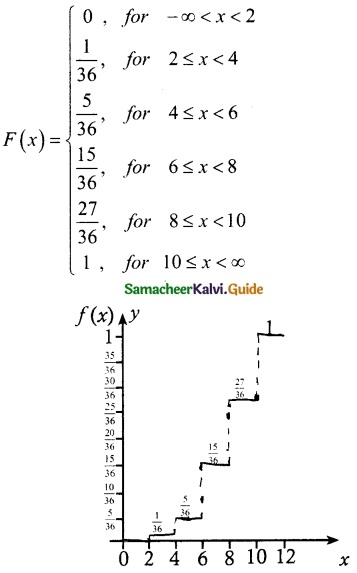Question 3.
Find the probability mass function and cumulative distribution function of a number of girl children in families with 4 children, assuming equal probabilities for boys and girls.
Solution:
Let X be the random variable denotes the number of girl child among 4 children
X = {0, 1, 2, 3, 4}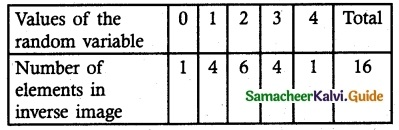(i) Probability mass function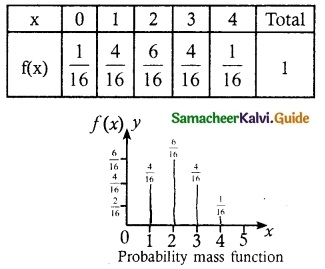(ii) Cumulative distribution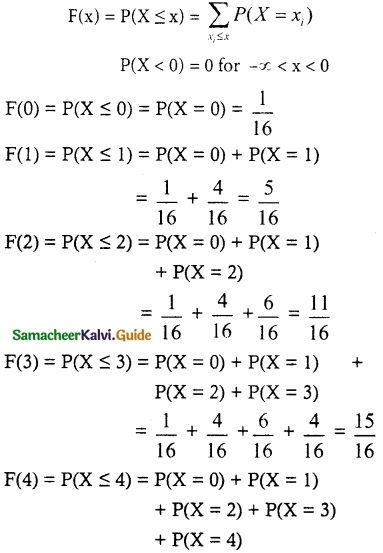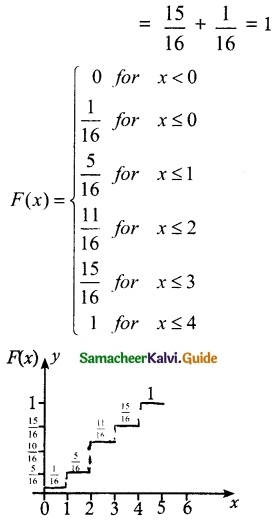Question 4.
Suppose a discrete random variable can only take the values 0, 1, and 2. The probability mass function is defined by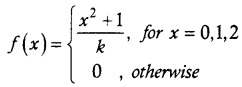Find (i) the value of k.
(ii) cumulative distribution function
(iii) P(X ≥ 1)
Solution: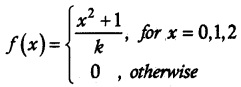(i) Given f is a probability mass function
$$\sum_{x}$$ f(x) = 1
Probability mass function is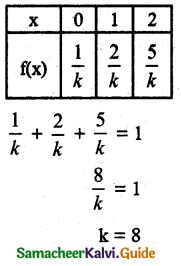(ii) Cumulative distribution function
F(0) = P(x ≤ 0)
= P(x = 0)
= $$\frac { 1 }{ 8 }$$
F(1) = P(x ≤ 1)
= P(x = 0) + P(x = 1)
= $$\frac { 1 }{ 8 }$$ + $$\frac { 2 }{ 8 }$$ = $$\frac { 3 }{ 8 }$$
F(2) = P(x ≤ 2)
= P(x = 0) + P(x = 1) + P(x = 2)
= $$\frac { 1 }{ 8 }$$ + $$\frac { 2 }{ 8 }$$ + $$\frac { 5 }{ 8 }$$ = 1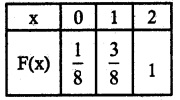(iii) P(X ≥ 1)
= P(X = 1) + P(X = 2)
= $$\frac { 2 }{ 8 }$$ + $$\frac { 5 }{ 8 }$$ + $$\frac { 7 }{ 8 }$$Question 5.
The cumulative distribution function of a discrete random variable is given by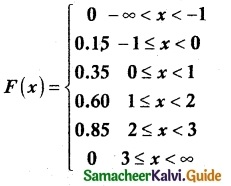Find
(i) the probability mass function
(ii) P(X < 1)
(iii) P(X ≥ 2)
Solution: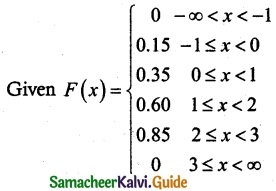f(-1) = P(X = -1) = F(-1) – F(-1) = 0.15 – 0 = 0.15
f(0) = P(X = 0) = F(0) – F(-1) = 0.35 – 0.15 = 0.20
f(1) = P(X = 1) = F(1) – F(0) = 0.60 – 0.35 = 0.25
f(2) = P(X = 2) = F(2) – F(1) = 0.85 – 0.60 = 0.25
f(3) = P(X = 3) = F(3) – F(2) = 1 – 0.85 = 0.15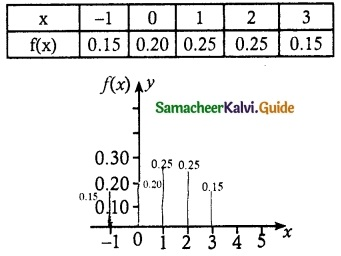(ii) P(X < 1)
P(X < 1) = P(X = -1) + P(X = 0)
= 0.15 + 0.20
= 0.35

(iii) P(X ≥ 2)
P(X ≥ 2) = P(X = 2) + P(X = 3)
= 0.25 + 0.15
= 0.40Question 6.
A random variable X has the following probability mass function.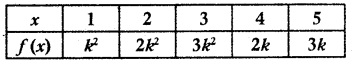Find
(i) the value of k
(ii) P(2 ≤ X < 5)
(iii) P(3 < X)
Solution:
(i) Given f(x) in a probability mass function
$$\sum_{x}$$ f(x) = 1
k² + 2k² + 3k² + 2k + 3k = 1
6k² + 5k = 1
6k² + 5k – 1 = 0
(k + 1) (6k – 1) = 0
k = $$\frac { 1 }{ 6 }$$
(k ≠ -1 neglecting negative terms)
Probability mass function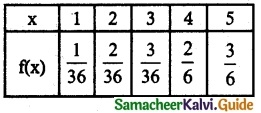(ii) P(2 ≤ X < 5)
P(2 ≤ X < 5) = P(X = 2) + P(X = 3) + P(X = 4)
= $$\frac { 2 }{ 36 }$$ + $$\frac { 3 }{ 36 }$$ + $$\frac { 2 }{ 6 }$$
= $$\frac { 2+3+12 }{ 36 }$$ + $$\frac { 17 }{ 36 }$$

(iii) P(X > 3) = P(X = 4) + P(X = 5)
= $$\frac { 2 }{ 6 }$$ + $$\frac { 3 }{ 6 }$$
= $$\frac { 5 }{ 6 }$$Question 7.
The cumulative distribution function of a discrete random variable is given by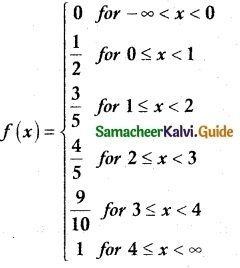Find
(i) the probability mass function
(ii) P(X < 3) and (iii) P(X ≥ 2)
Solution:
(i) Probability mass function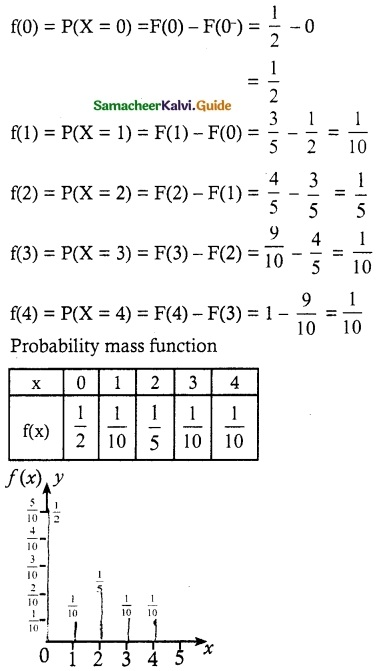(ii) P(X < 3)
P(X < 3) = P(X = 0) + P(X = 1) + P(X = 2)
= $$\frac { 1 }{ 2 }$$ + $$\frac { 1 }{ 10 }$$ + $$\frac { 1 }{ 5 }$$
= $$\frac { 4 }{ 5 }$$

(ii) P(X ≥ 2)
P(X ≥ 2) = P(X = 2) + P(X = 3) + P(X = 4)
= $$\frac { 1 }{ 5 }$$ + $$\frac { 1 }{ 10 }$$ + $$\frac { 1 }{ 10 }$$
= $$\frac { 4 }{ 10 }$$ = $$\frac { 2 }{ 5 }$$